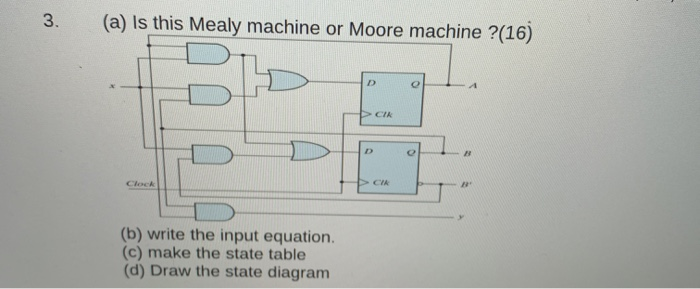# Question 3. (a) Is this Mealy machine or Moore machine ?(16) (b) write the input equation. (c) make the state table (d) Draw the state diagramFJA48Y The Asker · Computer ScienceTranscribed Image Text: 3. (a) Is this Mealy machine or Moore machine ?(16) (b) write the input equation. (c) make the state table (d) Draw the state diagram
More
Transcribed Image Text: 3. (a) Is this Mealy machine or Moore machine ?(16) (b) write the input equation. (c) make the state table (d) Draw the state diagram